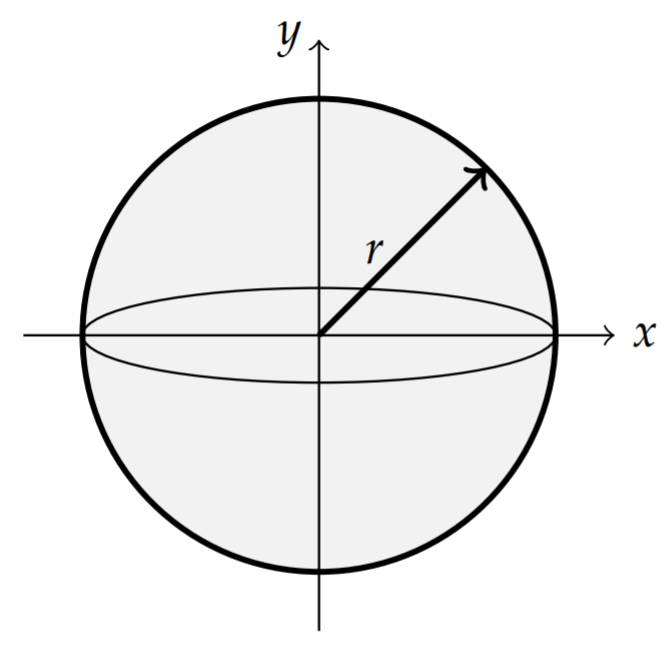# 6.6: Spherically Symmetric Vibrations

$$\newcommand{\vecs}{\overset { \rightharpoonup} {\mathbf{#1}} }$$ $$\newcommand{\vecd}{\overset{-\!-\!\rightharpoonup}{\vphantom{a}\smash {#1}}}$$$$\newcommand{\id}{\mathrm{id}}$$ $$\newcommand{\Span}{\mathrm{span}}$$ $$\newcommand{\kernel}{\mathrm{null}\,}$$ $$\newcommand{\range}{\mathrm{range}\,}$$ $$\newcommand{\RealPart}{\mathrm{Re}}$$ $$\newcommand{\ImaginaryPart}{\mathrm{Im}}$$ $$\newcommand{\Argument}{\mathrm{Arg}}$$ $$\newcommand{\norm}{\| #1 \|}$$ $$\newcommand{\inner}{\langle #1, #2 \rangle}$$ $$\newcommand{\Span}{\mathrm{span}}$$ $$\newcommand{\id}{\mathrm{id}}$$ $$\newcommand{\Span}{\mathrm{span}}$$ $$\newcommand{\kernel}{\mathrm{null}\,}$$ $$\newcommand{\range}{\mathrm{range}\,}$$ $$\newcommand{\RealPart}{\mathrm{Re}}$$ $$\newcommand{\ImaginaryPart}{\mathrm{Im}}$$ $$\newcommand{\Argument}{\mathrm{Arg}}$$ $$\newcommand{\norm}{\| #1 \|}$$ $$\newcommand{\inner}{\langle #1, #2 \rangle}$$ $$\newcommand{\Span}{\mathrm{span}}$$$$\newcommand{\AA}{\unicode[.8,0]{x212B}}$$

Another application of spherical harmonics is a vibrating spherical membrane, such as a balloon. Just as for the two-dimensional membranes encountered earlier, we let $$u(\theta, \phi, t)$$ represent the vibrations of the surface about a fixed radius obeying the wave equation, $$u_{t t}=c^{2} \nabla^{2} u$$, and satisfying the initial conditions $u(\theta, \phi, 0)=f(\theta, \phi), \quad u_{t}(\theta, \phi, 0)=g(\theta, \phi) .\nonumber$ In spherical coordinates, we have (for $$\rho=r=$$ constant.) $u_{t t}=\frac{c^{2}}{r^{2}}\left(\frac{1}{\sin \theta} \frac{\partial}{\partial \theta}\left(\sin \theta \frac{\partial u}{\partial \theta}\right)+\frac{1}{\sin ^{2} \theta} \frac{\partial^{2} u}{\partial \phi^{2}}\right),\label{eq:1}$ where $$u=u(\theta, \phi, t)$$.Figure $$\PageIndex{1}$$: A vibrating sphere of radius $$r$$ with the initial conditions \begin{aligned} &u(\theta, \phi, 0)=f(\theta, \phi), \\ &u_{t}(\theta, \phi, 0)=g(\theta, \phi) . \end{aligned}

The boundary conditions are given by the periodic boundary conditions $u(\theta, 0, t)=u(\theta, 2 \pi, t), \quad u_{\phi}(\theta, 0, t)=u_{\phi}(\theta, 2 \pi, t),\nonumber$ where $$0<t$$, and $$0<\theta<\pi$$, and that $$u=u(\theta, \phi, t)$$ should remain bounded.

Noting that the wave equation takes the form $u_{t t}=\frac{c^{2}}{r^{2}} L u, \quad \text { where } \quad L Y_{\ell m}=-\ell(\ell+1) Y_{\ell m}\nonumber$ for the spherical harmonics $$Y_{\ell m}(\theta, \phi)=P_{\ell}^{m}(\cos \theta) e^{i m \phi}$$, then we can seek product solutions of the form $u_{\ell m}(\theta, \phi, t)=T(t) Y_{\ell m}(\theta, \phi) .\nonumber$ Inserting this form into the wave equation in spherical coordinates, we find $T^{\prime \prime} Y_{\ell m}=-\frac{c^{2}}{r^{2}} T(t) \ell(\ell+1) Y_{\ell m},\nonumber$ or $T^{\prime \prime}+\ell(\ell+1) \frac{c^{2}}{r^{2}} T(t)\nonumber$ The solutions of this equation are easily found as $T(t)=A \cos \omega_{\ell} t+B \sin \omega_{\ell} t, \quad \omega_{\ell}=\sqrt{\ell(\ell+1)} \frac{c}{r} .\nonumber$ Therefore, the product solutions are given by $u_{\ell m}(\theta, \phi, t)=\left[A \cos \omega_{\ell} t+B \sin \omega_{\ell} t\right] Y_{\ell m}(\theta, \phi)\nonumber$ for $$\ell=0,1, \ldots, m=-\ell,-\ell+1, \ldots, \ell$$.Figure $$\PageIndex{2}$$: Modes for a vibrating spherical membrane: \begin{aligned}\text{Row }1:& (1,0), (1,1); \\ \text{Row }2:& (2,0), (2,1), (2,2); \\ \text{Row }3:& (3,0), (3,1), (3,2), (3,3).\end{aligned}

The general solution is found as $u(\theta ,\phi ,t)=\sum\limits_{\ell=0}^\infty\sum\limits_{m=-\ell}^{\ell}[A_{\ell m}\cos\omega_{\ell}t+B_{\ell m}\sin\omega_{\ell}t]Y_{\ell m}(\theta ,\phi ).\nonumber$ An interesting problem is to consider hitting the balloon with a velocity impulse while at rest. An example of such a solution is shown in Figure $$\PageIndex{3}$$. In this images several modes are excited after the impulse.Figure $$\PageIndex{3}$$: A moment captured from a simulation of a spherical membrane after hit with a velocity impulse.

This page titled 6.6: Spherically Symmetric Vibrations is shared under a CC BY-NC-SA 3.0 license and was authored, remixed, and/or curated by Russell Herman via source content that was edited to the style and standards of the LibreTexts platform; a detailed edit history is available upon request.